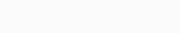# Linear Discriminant Analysis in Machine Learning with Python

Some datasets have thousands of features that give more information about data and that’s good but it takes large space and more time for computation of processing. For reducing the data storage and computation time use dimensionality reduction and get only relevant features. Let’s understand one of the techniques for dimensionality reduction that is linear discriminant analysis.

## Linear Discriminant Analysis (LDA)

The linear discriminant analysis is a technique for dimensionality reduction. Linear discriminant analysis (LDA) very similar to Principal component analysis (PCA). LDA is a form of supervised learning and gets the axes that maximize the linear separability between different classes of the data. LDA removes the variables that are not independent or important also removes variables derived from a combination of other variables for example if there are x variables than LDA decreases the variable to y variables to decrease the dimensionality. The removed variables are the combination of these y variables. These y variables are sufficient to separate classes of data. By reducing the variables dimensionality gets reduced and gets separate classes.

## Implementation of LDA in Python using Machine learning

We implement the LDA in python in three steps.

#### Step-1 Importing libraries

Here, we use libraries like Pandas for reading the data and transforming it into useful information, Scikit-Learn for LDA.

```import pandas as pd
from sklearn.model_selection import train_test_split
from sklearn.discriminant_analysis import LinearDiscriminantAnalysis
from sklearn.metrics import accuracy_score
from sklearn.preprocessing import StandardScaler
from sklearn.ensemble import RandomForestClassifier```

```iris=pd.read_csv("Iris.csv")
iris=iris.drop('Id',axis=1)

Output:-Step-3 Performing Linear discriminant analysis

Getting input and target from data.

```X=iris.drop('Species',axis=1)
y=iris['Species']```

Splitting data into test and train data.

`X_train,X_test,y_train,y_test=train_test_split(X,y,test_size=0.3,random_state=37)`

We use standard scalar to get optimum results.

```SC=StandardScaler()
X_train = SC.fit_transform(X_train)
X_test = SC.transform(X_test)```

Defining and performing LDA.

```LDA = LinearDiscriminantAnalysis(n_components=1)
X_train = LDA.fit_transform(X_train, y_train)
X_test = LDA.transform(X_test)```

To classify we use the Random forest classifier.

```RFC = RandomForestClassifier(max_depth=3, random_state=34)
RFC.fit(X_train, y_train)```

Predicting the species.

`pred=RFC.predict(X_test)`

Calculating the accuracy.

`accuracy_score(y_test,pred)`

Output:-Dataset

Here, we are using the Iris dataset. It contains the data of three species and its sepal length, sepal width, petal length, petal width. You can download the dataset from here: https://archive.ics.uci.edu/ml/datasets/iris

#### Conclusion

Here we see the followings:

• Linear discriminant analysis(LDA)
• Implementation of LDA in python.